Definitions of Square Dance Calls and Concepts

Index -->  Plus  |  A1  |  A2  |  C1  |  C2  |  C3A  |  C3B  |  C4  |  NOL  |
Definitions (Text Only) -->  Plus  |  A1  |  A2  |  C1  |  C2  |  C3A  |  C3B  |  C4  |  NOL  |
 Find call:

Oddly any CONCEPT anything CONCEPT [C3B]:
anything call \$B\$r%Q!<%H\$KJ,2r\$7(B, \$B4q?t\$N%Q!<%H\$O(B any Concept \$B\$G9T\$\$(B, \$B6v?t\$N%Q!<%H\$OIaDL\$K9T\$\$\$^\$9(B.
\$BLu

Evenly any CONCEPT anything CONCEPT [C3B]:
anything call \$B\$r%Q!<%H\$KJ,2r\$7(B, \$B6v?t\$N%Q!<%H\$O(B any Concept \$B\$G9T\$\$(B, \$B4q?t\$N%Q!<%H\$OIaDL\$K9T\$\$\$^\$9(B.
\$BLu

\$B%Q!<%H\$KJ,\$1\$i\$l\$k%3!<%k\$O(B, \$B;dC#\$N(B Definitions \$BA4BN\$G<(\$5\$l\$F\$\$\$^\$9(B. \$BCalls with Parts.

\$BNc\$(\$P(B, Oddly Tandem Swing & Mix \$B\$O(B Tandem Swing, \$BIaDL\$K(B Centers Cross Run, Tandem Centers Trade \$B\$G\$9(B.

Oddly Tandem Remake: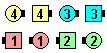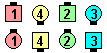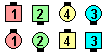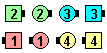Oddly Tandem Remake\$B\$NA0(B Tandem Right Arm Turn 1/4\$B\$N8e(B (normal) Left Arm Turn 1/2\$B\$N8e(B Tandem Right Arm Turn 3/4\$B\$N8e(B (\$B=*\$o\$j(B)Oddly Single Grand Cross Trade & Wheel\$B\$NA0(B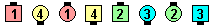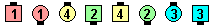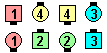Single As Couples Hinge\$B\$N8e(B (normal) Center 6 Trade\$B\$N8e(B Single As Couples Step & Fold\$B\$N8e(B (\$B=*\$o\$j(B)Oddly Once Removed Reset\$B\$NA0(B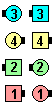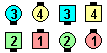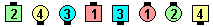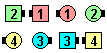Once Removed 1/2 Zoom\$B\$N8e(B (normal) Hinge\$B\$N8e(B Once Removed 1/2 Zoom\$B\$N8e(B (normal) Hinge\$B\$N8e(B (\$B=*\$o\$j(B)
Evenly Tandem Reset:Evenly Tandem Reset\$B\$NA0(B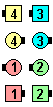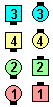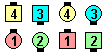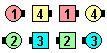1/2 Zoom\$B\$N8e(B Tandem Hinge\$B\$N8e(B 1/2 Zoom\$B\$N8e(B Tandem Hinge\$B\$N8e(B (\$B=*\$o\$j(B)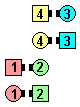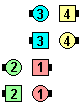Evenly As Couples Pass "Z" Axlet\$B\$NA0(B Pass Thru\$B\$N8e(B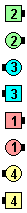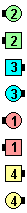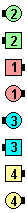As Couples Ends Cross Cast Back\$B\$N8e(B (normal) Trade\$B\$N8e(B As Couples Centers Trade\$B\$N8e(B (\$B=*\$o\$j(B)

\$BNr;KE*\$K(B,
Oddly \$B\$O(B Random \$B\$H\$7\$FCN\$i\$l\$F\$-\$^\$7\$?(B, \$B\$=\$7\$F(B
Evenly \$B\$O(B Reverse Random \$B\$H\$7\$FCN\$i\$l\$F\$-\$^\$7\$?(B.

Randomize Between any CONCEPT And any CONCEPT anything [C4] : \$B4q?t\$N%Q!<%H\$O;O\$a\$KM?\$(\$i\$l\$?(B CONCEPT \$B\$G9T\$\$(B, \$B6v?t\$N%Q!<%H\$OONCEPT \$B\$G9T\$\$\$^\$9(B.

\$B

• Initially CONCEPT [C3A]
• Finally CONCEPT [C3A]
•Choreography for Oddly | Evenly ConceptComments? Questions? Suggestions?

https://www.ceder.net/def/random.php?language=japan
08-August-2020 11:35:56